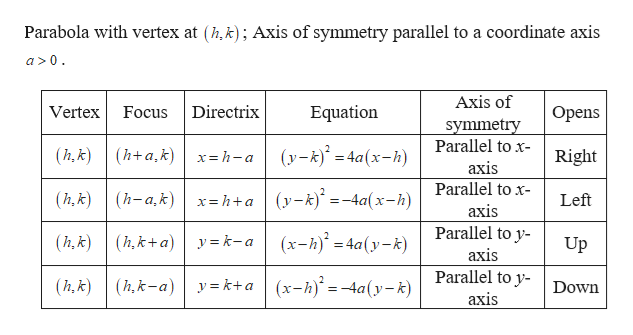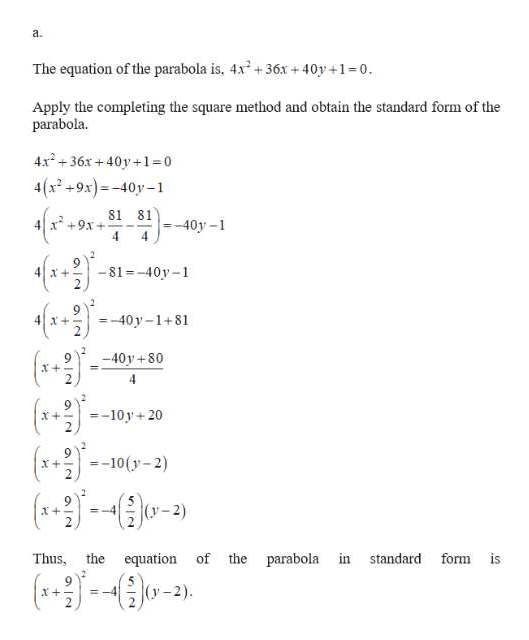# For Exercise, an equation of a parabola is given.a. Write the equation of the parabola in standard form.\b. Identify the vertex, focus, and focal diameter. 4x2 + 36x + 40y + 1=0

Question
1 views

For Exercise, an equation of a parabola is given.

a. Write the equation of the parabola in standard form.

\b. Identify the vertex, focus, and focal diameter. 4x2 + 36x + 40y + 1=0

check_circle

Step 1help_outlineImage TranscriptioncloseParabola with vertex at (h,k); Axis of symmetry parallel to a coordinate axis a>0. Axis of Directrix Equation Opens Vertex Focus symmetry Parallel to x- axis Parallel to x- (y-k)° = 4a(x-h) (h, k) (h+a,k) Right x= h-a x= h+a (y-k)* =-4a(x-h) (h, k) (h-a,k) Left axis Parallel to y- (x-h) = 4a(y-k) (h, k)| (h,k+a) | y = k-a Up axis Parallel to y- y = k+a (x-h)* =-4a(y-k) (h,k) (h,k-a) Down axis fullscreen
Step 2help_outlineImage Transcriptionclosea. The equation of the parabola is, 4x + 36x + 40y +1=0. Apply the completing the square method and obtain the standard form of the parabola. +36x +40y +1=0 4(x +9x)=-40y-1 81 81 =-40y -1 4 x* +9x +--- %3D - 81 =-40y -1 =-40 y-1+81 -40y +80 =-10 y + 20 X+ (*+)- x--10(y- 2) v-2) (y =-4 parabola in standard form is Thus, the equation of the (y-2). = -4 fullscreen

### Want to see the full answer?

See Solution

#### Want to see this answer and more?

Solutions are written by subject experts who are available 24/7. Questions are typically answered within 1 hour.*

See Solution
*Response times may vary by subject and question.
Tagged in

### Other